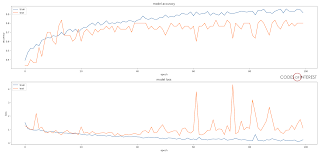# How to Graph Model Training History in Keras

When we are training a machine learning model in Keras, we usually keep track of how well the training is going (the accuracy and the loss of the model) using the values printed out in the console. Wouldn't it be great if we can visualize the training progress? Not only would it be easier to see how well the model trained, but it would also allow us to compare models.

Something like this?Training accuracy and loss for 100 epochs

Well, you can actually do it quite easily, by using the History objects of Keras along with Matplotlib.

When you are using model.fit() to train a model (or, model.fit_generator() when using a generator) it actually return a History object.

`````` history = model.fit(train_data, train_labels,
nb_epoch=100, batch_size=32,
validation_data=(validation_data, validation_labels))
``````

In this History object the History.history attribute contains training accuracy and loss, as well as the validation accuracy and loss, for each of the training epochs. You can check what matrices are available in the History object by printing out the keys of History.history.
`````` print(history.history.keys())
``````

Which will print,
`````` ['acc', 'loss', 'val_acc', 'val_loss']
``````

Note: The 'val_acc' and 'val_loss' keys will only be present if you use the 'validation_data' parameter in mode.fit(). Otherwise, the history object will only contain 'acc' and 'loss'.

With all the matrices at hand, now we can plot them.

We use Matplotlib for that. We need to plot 2 graphs: one for training accuracy and validation accuracy, and another for training loss and validation loss. Since the show() function of Matplotlib can only show one plot window at a time, we will use the subplot feature in Matplotlibto draw both the plots in the same window.

`````` ...
...
import matplotlib.pyplot as plt

...
...
# code for building your model
...
...

history = model.fit(train_data, train_labels,
nb_epoch=nb_epoch, batch_size=32,
validation_data=(validation_data, validation_labels))

print(history.history.keys())

plt.figure(1)

# summarize history for accuracy

plt.subplot(211)
plt.plot(history.history['acc'])
plt.plot(history.history['val_acc'])
plt.title('model accuracy')
plt.ylabel('accuracy')
plt.xlabel('epoch')
plt.legend(['train', 'test'], loc='upper left')

# summarize history for loss

plt.subplot(212)
plt.plot(history.history['loss'])
plt.plot(history.history['val_loss'])
plt.title('model loss')
plt.ylabel('loss')
plt.xlabel('epoch')
plt.legend(['train', 'test'], loc='upper left')
plt.show()
``````